# coversim

## Introduction

The coversim function performs coverage simulations for two-dimensional confidence region plots, and is part of the conf package. It is capable of performing numerous simulation iterations (or replications) in a single command.

Each trial within the coversim function uses a random dataset with user-specified parameters (default), or a user specified dataset matrix. Each trial identifies if a point of interest (either the population parameters or a user specified point) is within or outside of the confidence region.

The coversim function returns the number of replications completed, replications containing the point of interest within the confidence region, and if any replications resulted in an error. Errors are uncommon but occur when crplot is unable to plot the confidence region, typically due challenging confidence region size (when alpha ~ 0) and/or shape (small sample sizes, e.g., two or three samples to estimate a two-parameter univariate probability model).

## Methodology

The coversim function calls the crplot function (also in the conf package) in each of its trials. The crplot function plots a confidence region corresponding to the random (default) or user specified dataset (using the optional argument dataset). It then leverages the inpolygon function from the pracma package to determine if the confidence region covers or misses the point of interest (meaning the point is or is not contained within its enclosed area). It prints a summary of the results (replications by total number, covered, and errors) to the console upon completion, with the option to return and store additional data.

## Installation Instructions

The coversim function is accessible following installation of the conf package:

install.packages("conf")
library(conf)

## Examples: a single coversim trial

The crplot function is first shown below before demonstrating the coversim function. The confidence region for 10 random variates from a normal$$(\mu = 5, \sigma = 10)$$ is plot using:

library(conf)
set.seed(1)
crplot(rnorm(10, mean = 5, sd = 10), alpha = 0.1, distn = "norm")
#>  "90% confidence region plot complete; made using 98 boundary points."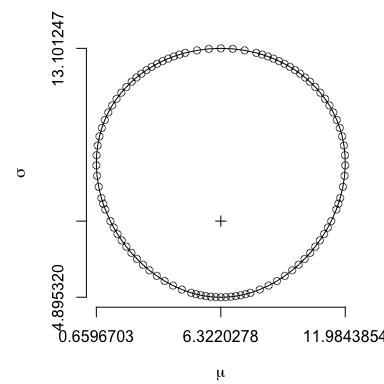Shown next, the coversim function both completes a confidence region plot and identifies if its area contains the true population parameters (as its default) in a single command. The population parameters are specified in coversim using their Greek alphabet symbol name. Use ?coversim to view the help page containing probability density functions corresponding to each available distribution in order to ensure proper usage.

coversim(alpha = 0.1, distn = "norm", n = 10, iter = 1, mu = 5, sigma = 10, showplot = TRUE, seed = 1)
#>  "------------------ 10 samples in each of 1 iterations ---------------------"
#>
#>  "...now using alpha: 0.1"
#>
100% complete
#>  "1 replications complete."
#>  "1 confidence regions contained the true parameters."
#>  "0 total errors."
#>
#>  "coverage simulation summary (1 replications per parameterization):"
#>      alpha  n coverage errors
#> [1,]   0.1 10        1      0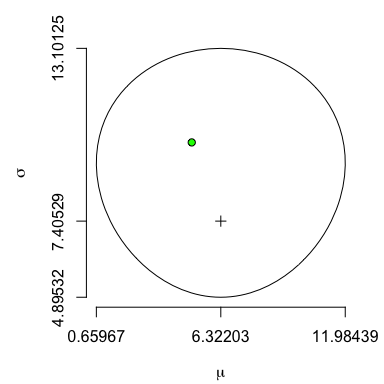Note that an identical confidence region area results in the two preceding plots because the random number stream in both is set using the optional coversim argument seed = 1. The optional argument showplot is also set to TRUE so that the resulting confidence region plot is shown. Its plot is augmented with a green point at the population parameters, $$(\mu = 5, \sigma = 10)$$, indicating it is contained within the confidence region area.

It is also possible to identify coverage (or lack thereof) of a point other than the population parameters using coversim. This is done with the optional argument point, set to the chosen coordinate. Next, the point $$(\mu = 10, \sigma = 6)$$ is assessed using the same confidence region. That point just misses coverage within its confidence region area, therefore coversim colors both the point and its corresponding confidence region boundary red to indicate missed coverage.

coversim(alpha = 0.1, distn = "norm", n = 10, iter = 1, mu = 5, sigma = 10, showplot = TRUE, seed = 1, point = c(10, 6))
#>  "------------------ 10 samples in each of 1 iterations ---------------------"
#>
#>  "...now using alpha: 0.1"
#>
100% complete
#>  "1 replications complete."
#>  "0 confidence regions contained the true parameters."
#>  "0 total errors."
#>
#>  "coverage simulation summary (1 replications per parameterization):"
#>      alpha  n coverage errors
#> [1,]   0.1 10        0      0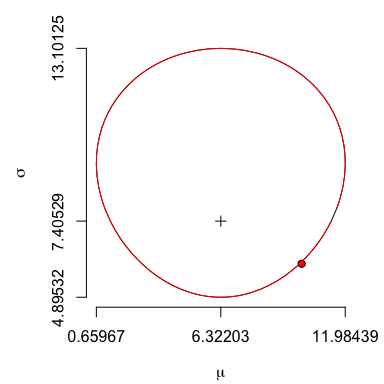## Examples: multiple coversim iterations

The coversim function can automate and record coverage assessments for numerous trials in a single command. The example below illustrates 10 iterations, each developing a confidence region from 16 random sample values from a gamma$$(\theta = 1, \kappa = 2)$$ distribution.

par(mfrow = c(2, 5))
coversim(alpha = 0.5, distn = "gamma", n = 16, iter = 10, theta = 1, kappa = 2, showplot = TRUE, mlelab = FALSE, xlim = c(0, 2), ylim = c(0, 4.5), sf = c(1, 2), ylas = 1)
#>  "------------------ 16 samples in each of 10 iterations ---------------------"
#>
#>  "...now using alpha: 0.5"
#>
10% complete
20% complete
30% complete
40% complete
50% complete
60% complete
70% complete
80% complete
90% complete
100% complete
#>  "10 replications complete."
#>  "6 confidence regions contained the true parameters."
#>  "0 total errors."
#>
#>  "coverage simulation summary (10 replications per parameterization):"
#>      alpha  n coverage errors
#> [1,]   0.5 16      0.6      0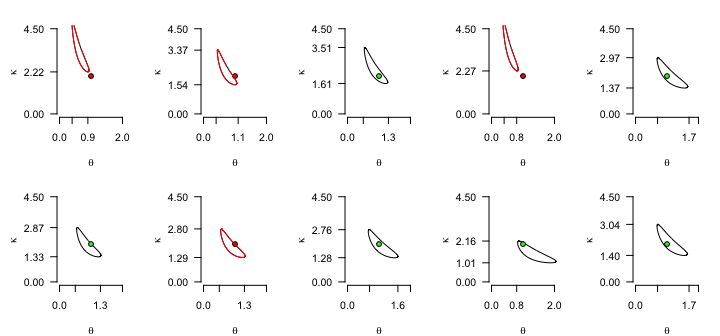Both the console output and the plots indicate that 6 of 10 iterations produce confidence regions covering the true population parameters, and 4 of 10 iterations result in confidence regions that miss the population parameters. The actual coverage for this simulation is therefore 0.6, whereas its nominal (or stated) coverage is 0.5.

For likelihood-ratio based confidence regions of two-parameter univariate probability models, the nominal coverage is asymptotically exact. For small sample sizes, however, typically a negative bias exists (albeit counter to the aforementioned result attained using only 10 iterations). The next simulation will support this claim.

Simulate a $$90\%$$ confidence region for $$n = \{2, 3, \ldots, 30\}$$ random samples from a Weibull$$(\kappa = 3, \lambda = 1 / 2)$$ population. For each $$n$$, conduct $$10,000$$ replications, and report its resulting actual coverage.

# Note: due to its long runtime, plot results pictured below were imported.  Code producing analogous (but not identical) results is none-the-less given here:
reps <- 10000      # 10,000 iterations per (alpha, n) parameterization
n <- c(2:30)       # sample sizes to assess
coversim(alpha = 0.1, distn = "weibull", n = n, iter = reps, kappa = 3, lambda = 1/2, main = "Weibull(kappa = 3, lambda = 0.5) \n Results at 90% Nominal Coverage \n (iter = 10,000 per datapoint)")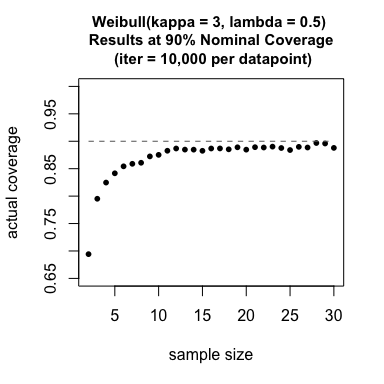Notable separation between the nominal (dotted line) and actual coverages (points) for small $$n$$ supports the claim of negative bias for confidence region coverage at those respective sample sizes for this particular population probability distribution.

The coversim command above provides a summary plot of sample size vs actual coverage because length(n) > length(alpha). When length(n) < length(alpha), summary plot(s) of nominal coverage vs actual coverage result. The next plot demonstrates those circumstances.

# Note: due to its long runtime, plot results pictured below were imported (patched together from several days of recording).  Code producing analogous (but not identical) results is none-the-less given here:
reps <- 10000         # 10,000 iterations per parameterization
n <- 100              # sample sizes to assess
a <- seq(0.1, 0.9, by = 0.01)   # alpha values to assess
coversim(alpha = a, distn = "weibull", n = n, iter = reps, kappa = 3, lambda = 1/2, main = "Weibull(kappa = 3, lambda = 0.5) Coverage \n Results for n = 100 (iter = 10,000 per datapoint)")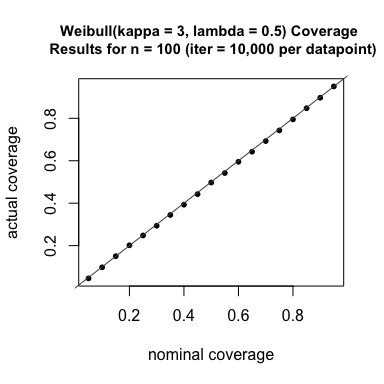This plot demonstrates that $$n = 100$$ samples result in a confidence region whose actual coverage probability is close to its nominal coverage. This is an intuitive result considering its likelihood-ratio based confidence region is asymptotically chi-square distributed; nominal and actual coverage converge as the sample size increases.

## Custom graphics and analysis using info = TRUE

The optional argument info = TRUE directs coversim to return a list summarizing all simulations. It will print to the console, unless directed via x <- coversim(..., info = TRUE) to store in x (or whatever name specified). The latter is recommended.

The returned list has the following labels (with accompanying descriptions):

• alab: a vector associated with alpha values for the corresponding results
• nlab: a vector associated with n values (sample size) for the corresponding results
• results: a matrix; rows represent unique (alab, nlab) parameterization, columns represent coverage results per iteration (1 if covered, 0 otherwise)
• errors: a matrix; rows represent unique (alab, nlab) parameterization, columns represent iteration error results (1 if error, 0 otherwise)
• coverage: a vector associated with (# covered) / (# successfully assessed), where the quantity successfully assessed ignores any iteration with a confidence region plot error

Additional information is returned when the following optional arguments are set to TRUE:

• samples (returned using the optional argument returnsamp = TRUE): a matrix of $$n$$ rows and iter columns containing the random samples used in the last parameterization of the simulation (corresponding to its last parameterization if multiple are used)
• quantiles (returned using the optional argument returnquant = TRUE): a matrix of $$n$$ rows and iter columns containing the cdf value of corresponding to the random samples (corresponding to its last parameterization if multiple are used)

The next example uses info = TRUE to customize its results, combining and coloring graphics to facilitate their comparison. The next section details how to customize plots such as this one using the info = TRUE optional argument.

# Note: due to its long runtime, plot results pictured below were imported (patched together from several days of recording).  Code producing analogous (but not identical) results is none-the-less given here:
reps <- 10000                       # 10,000 iterations per parameterization
n1 <- c(3, 5, 10)                   # sample sizes to assess
a1 <- seq(0.99, 0.01, by = -0.01)   # alpha values to assess n = c(3, 5, 10)
a2 <- seq(0.9,  0.1,  by = -0.1)    # alpha values to assess n = 100
x1 <- coversim(alpha = a1, distn = "weibull", n = n1,  iter = reps, kappa = 3, lambda = 1/2, info = TRUE)
x2 <- coversim(alpha = a2, distn = "weibull", n = 100, iter = reps, kappa = 3, lambda = 1/2, info = TRUE)
index3 <- which(x1$nlab == 3) index5 <- which(x1$nlab == 5)
index10 <- which(x1$nlab == 10) # make a custom plot with results stored in the lists x1 and x2 par(xpd = TRUE) plot(1 - x2$alab, x2$coverage, xlim = c(0, 1), ylim = c(0, 1), axes = FALSE, pch = 16, cex = 0.3, col = "forestgreen", xlab = "nominal coverage", ylab = "actual coverage") title(main = "Weibull(kappa = 3, lambda = 0.5) \n Coverage Results for Various Sample \n Sizes and Nominal Coverages \n (iter = 10,000 per datapoint)", cex.main = 0.92) points(1-x1$alab[index3], x1$coverage[index3], col = "red", pch = 16, cex = 0.3) # n = 3 points(1-x1$alab[index5], x1$coverage[index5], col = "orange", pch = 16, cex = 0.3) # n = 5 points(1-x1$alab[index10], x1\$coverage[index10], col = "blue", pch = 16, cex = 0.3) # n = 10
lines(c(0, 1), c(0, 1), lty = 1, col = "gray70")
axis(side = 1, at = seq(0, 1, by = 0.2), labels = TRUE)
axis(side = 2, at = seq(0, 1, by = 0.2), labels = TRUE)
legend(0.02, 0.98, legend = rev(c("n = 100", "n = 10", "n = 5", "n = 3", "nominal coverage = actual coverage")), pch = rev(c(16, 16, 16, 16, NA)), col = c("black", "red", "orange", "blue", "forestgreen"), lty = rev(c(NA, NA, NA, NA, 1)), cex = 0.5, y.intersp = 1.5, box.col = NA, bg = NA, pt.lwd = 0.4)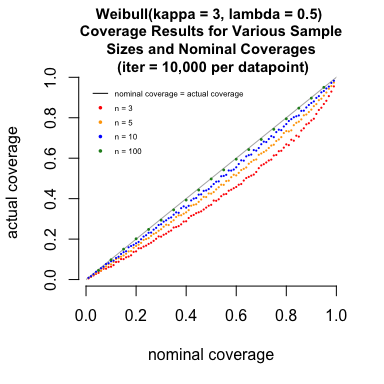Customizing this plot successfully highlights the difference of results between the various parameterizations of the simulation, providing useful intuition how actual coverage varies for small $$n$$ as a function of the nominal coverage.

## Coverage analysis of user defined datasets

The coversim function allows its user to provide their own sample, rather than drawing a random sample from a user-defined parametric population distribution. In those circumstances the user must provide either a vector (for a single iteration) or an n by iter matrix (for multiple iterations) of samples, and a point of interest to assess coverage relative to.

The next example illustrates a single coverage assessment for a user-defined dataset of ball bearing failure times, given by Lieblein and Zelen1, relative to a user-defined the point of interest. Its fit to the Weibull distribution is explained in depth in the Reliability textbook by Leemis2. After storing ball bearing failure times (in millions of revolutions) into the vector ballbearing, coversim assesses that a $$90\%$$ confidence region does not cover the point $$\kappa = 1, \lambda = 0.015$$. The fact this point (with $$\kappa = 1$$) is not covered supports the intuition that the ball bearings are wearing out, and therefore their times to failure do not follow an exponential distribution.

ballbearing <- c(17.88, 28.92, 33.00, 41.52, 42.12, 45.60, 48.48, 51.84,
51.96, 54.12, 55.56, 67.80, 68.64, 68.64, 68.88, 84.12,
93.12, 98.64, 105.12, 105.84, 127.92, 128.04, 173.40)
coversim(alpha = 0.1, distn = "weibull", dataset = ballbearing, point = c(1, 0.015), showplot = TRUE, origin = TRUE)
#>  "------------------ 23 samples in each of 1 iterations ---------------------"
#>
#>  "...now using alpha: 0.1"
#>
100% complete
#>  "1 replications complete."
#>  "0 confidence regions contained the true parameters."
#>  "0 total errors."
#>
#>  "coverage simulation summary (1 replications per parameterization):"
#>      alpha  n coverage errors
#> [1,]   0.1 23        0      0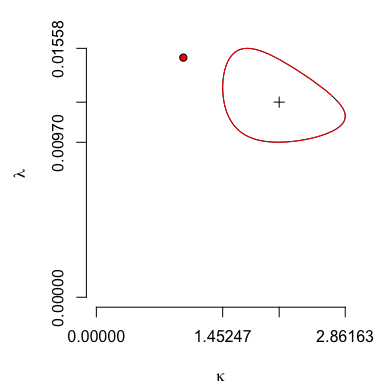The coversim function dataset argument can also accept multiple iterations of user-specified samples in a single command. For those circumstances, dataset is an n by iter matrix of sample values: each row representing one of n drawn samples, and each column representing a sample set. This capability is demonstrated next.

Suppose five samples of the aforementioned ball bearing dataset were each collected by four different engineers, and the $$90\%$$ confidence region of each respective subset is assessed for coverage of the point $$\kappa = 1, \lambda = 0.015$$. In this case dataset is a 5-row, 4-column matrix, with each column representing a sample set corresponding to one of the engineers. Each column of the dataset matrix produces a confidence region for the Weibull population parameters, which is subsequently assessed to either cover or miss the $$\kappa = 1, \lambda = 0.015$$ point of interest.

set.seed(1)              # ensure consistent results will illustrate in this vignette
par(mfrow = c(2, 2))     # display resulting plots in a 2 row by 2 column grid
samplematrix <- matrix(sample(ballbearing, 20), ncol = 4)   # subset 20 samples into four groups (columns)
coversim(alpha = 0.1, distn = "weibull", dataset = samplematrix, point = c(1, 0.015),
sf = c(2, 3), ylas = 1, showplot = TRUE, origin = TRUE)
#>  "------------------ 5 samples in each of 4 iterations ---------------------"
#>
#>  "...now using alpha: 0.1"
#>
25% complete
50% complete
75% complete
100% complete
#>  "4 replications complete."
#>  "0 confidence regions contained the true parameters."
#>  "0 total errors."
#>
#>  "coverage simulation summary (4 replications per parameterization):"
#>      alpha n coverage errors
#> [1,]   0.1 5        0      0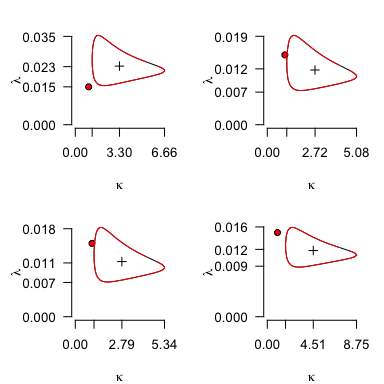Three of four $$90\%$$ confidence regions cover the point $$\kappa = 1, \lambda = 0.015$$. This is partially a consequence of reducing the sample size used to plot each confidence region (from $$23$$ to $$5$$), which in-turn increases uncertainty surrounding its maximum likelihood estimator (the area contained within the $$90\%$$ confidence region).

1. Lieblein, J., and Zelen, M. (1956), Statistical Investigation of the Fatigue Life of Deep-Groove Ball Bearings, Journal of Research of the National Bureau of Standards, 57, 273–316

2. Leemis, L. (1995), Reliability: Probabilistic Models and Statistical Methods, Prentice-Hall Inc., 345–251